## Ratio and Proportion For SBI PO : Set – 06

1) The largest and the second largest angles of a triangle are in the ratio of 7 : 3 respectively. The smallest angle is 20% of the sum of the largest and the second largest and the second largest angles. What is the sum of the smallest and the second largest angles?

a) 80

b) 60

c) 75

d) 90

e) None of these

c)

2) The incomes of two persons Ramesh and Arjun are in the ratio 5 : 8 and their expenditures are in the ratio 4: 7. If both the persons save equal amounts of Rs. 3000 each, what is the difference between their incomes?

a) Rs. 6000

b) Rs. 8000

c) Rs. 9000

d) Rs. 5000

e) None of these

c)

Income – saving = expenditure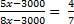35x – 3000 × 7 = 32x – 3000 × 4

3x = 3000 × 3

Difference => 8x – 5x => 3x = 9000

3) Two numbers are in the ratio 3:4. If 12 is added to each number they will be in the ratio 6:7. What is the product of these 2 numbers?

a) 192

b) 12

c) 42

d) 68

e) None of these

a)

Let the two numbers be 3x, 4x.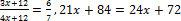x =4, 3x=12, 4x=16

Product of two numbers =12 × 16 =192

4) In a school the ratio of boys to girls is in the ratio 7:8 if there are 2400 students in the school then how many girls are in the school?

a) 2400

b) 1120

c) 1280

d) 1200

e) None of these

C)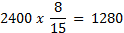5) Amul and Bala started a business with initial capital in the ratio 24:11 and their annual profit is in the ratio of 4:1. If Amul invested the money for 22 months Bala invested the money for?

a) 11 months

b) 9 months

c) 11 1/2 months

d) 12 months

e) None of these

d)

Capital * Time = Profit

24*22:11*x=4:1

528:11x=4:1

x= 12

Bala invested the money for 12 months

6) Rs 73,689 are divided between A and B in the ratio 4 : 7. What is the difference between thrice the share of A and twice the share of A and twice the share of B?

a) Rs. 36,699

b) Rs. 46,893

c) Rs. 20,097

d) Rs. 26,796

e) Rs. 13,398

e)

7) A certain amount was to be distributed among A, B and C in the ratio 2 : 3 : 4, but was erroneously distributed in the ratio 7 : 2 : 5. As a result of this, B got 740 less. What is the amount?

a) Rs 210

b) Rs 270

c) Rs 270

d)Rs 270

e) None of these

a)

8) Two mixtures contain tea and coffee in the ratio of 3:4 and 5:3 respectively. Find the ratio of the volumes of the quantities from the two mixtures to be mixed together so that the new mixture may contain same volume of Tea and coffee.

a) 7:4

b) 9: 6

c) 3:2

d) 7:8

e) None of these

a)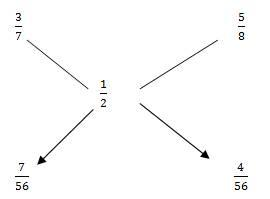9) The Weights of two friends Ram and shyam are in the ratio of 5:7. Ram’s weight increases by 20% and the total weight of Ram and shyam together becomes 82.8 kg., an increase of 27%. By what percent did the weight of shyam increase?

a) 19

b) 20

c) 15

d) 32

e) None of these

(d)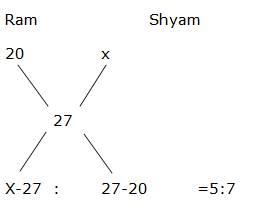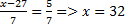10) The ratio of number of boys to the number of girls studying in a school is 25: 29. The total number of students studying in the school is 270. If 15 boys and 15 girls take admission in the school, what will be the new ratio of the boys and girls studying in the school?

a) 6: 7

b) 8: 9

c) 7: 8

d) 7: 9

e) None of these

(c)

The number of boy in school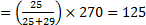The number of boy in school

= 270 – 125 = 145

Ratio => (125 + 15): (145 + 15)

140: 160 => 7: 8